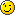This is an old revision of the document!

# Lab 10 - Machine Learning

## Objectives

• Understand basic concepts of machine learning
• Remember examples of real-world problems that can be solved with machine learning
• Learn the most common performance evaluation metrics for machine learning models
• Analyse the behaviour of typical machine learning algorithms using the most popular techniques
• Be able to compare multiple machine learning models

## Exercises

The exercises will be solved in Python, using various popular libraries that are usually integrated in machine learning projects:

• Scikit-Learn: fast model development, performance metrics, pipelines, dataset splitting
• Pandas: data frames, csv parser, data analysis
• NumPy: scientific computation
• Matplotlib: data plotting

All tasks are tutorial based and every exercise will be associated with at least one “TODO” within the code. Those tasks can be found in the exercises package, but our recommendation is to follow the entire skeleton code for a better understanding of the concepts presented in this laboratory class. Each functionality is properly documented and for some exercises, there are also hints placed in the code.

Because the various tasks and exercises are spread throughout the laboratory text, they are marked with a ⚠️ emoji. Make sure you look for this emoji so that you don't miss any of them!

Comment the results by specifying which is the best model in terms of fitting and which are the models that overfit or underfit the dataset.

### ⚠️ [15p] Exercise 5

In this exercise, you will learn how to properly evaluate a clustering model. We chose a K-means clustering algorithm for this example, but feel free to explore other alternatives. You can find out more about K-means clustering algorithms here. For all the associated tasks, you don't have to use any input file, because the clusters are generated in the skeleton. The model must learn how to group together points in a 2D space.

The solution for this exercise should be written in the TODO sections marked in the clustering.py file. Please follow the skeleton code and understand what it does. To run the code, uncomment perform_clustering() in app.py.

Compute the silhouette score of the model by using a Scikit-learn function found in the metrics package.

Fetch the centres of the clusters (the model should already have them ready for you) and plot them together with a colourful 2D representation of the data groups. Your plot should look similar to the one below:

You can also play around with the standard deviation of the generated blobs and observe the different outcomes of the clustering algorithm:

CLUSTERS_STD = 2

You should be able to discuss these observations with the assistant.

HINT: The plotting code is very similar to the one found in the skeleton. You can also Google it out.